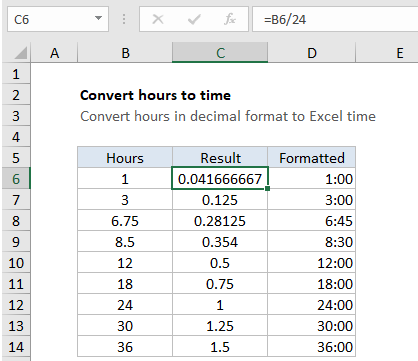## Excel Office

Excel How Tos, Tutorials, Tips & Tricks, Shortcuts

# Convert decimal hours to Excel time

This tutorial shows how to Convert decimal hours to Excel time using example below.

To convert hours in decimal format to a value Excel recognizes as time, divide by 24.

## Formula

`=hours/24`## Explanation

In the example shown the formula in C5 is:

`=B5/24`

which returns 0.04167, the equivalent of 1 hours. Cell D6 shows the same result formatted as time, which displays 1:00.

### How this formula works

In the Excel date system, one day is equal to 1, so you can think of time as fractional values of 1, as shown in the table below:

Hours Fraction Value Time
1 1/24 0.04167 1:00
3 3/24 0.125 3:00
6 6/24 0.25 6:00
4 4/24 0.167 4:00
8 8/24 0.333 8:00
12 12/24 0.5 12:00
18 18/24 0.75 18:00
21 21/24 0.875 21:00

This means if you have a decimal number for hours, you can simply divide by 24 to get the correct representation of hours in Excel. After dividing by 24, you can apply a time format of your choice, or use the result in a math operation with other dates or times.

In the example, since B10 contains 12 (representing 12 hours) the result is 12/24 = 0.5, since there are 12 hours in a half of day. Once a time format like h:mm has been applied, Excel will display 12:00.

### Durations longer than 24 hours

To display hours that represent a duration longer than 24 hours, you’ll need to adjust the number format. Just wrap the h in square brackets like so:

`[h]:mm`

To display in minutes, you can do the same thing with m:

`[m]`